Documentation

## Temperature Control in a Shower

This model shows how to implement a fuzzy inference system (FIS) in a Simulink® model.

The model controls the temperature of a shower using a fuzzy inference system implemented using a Fuzzy Logic Controller block. Open the `shower` model.

```open_system('shower') ```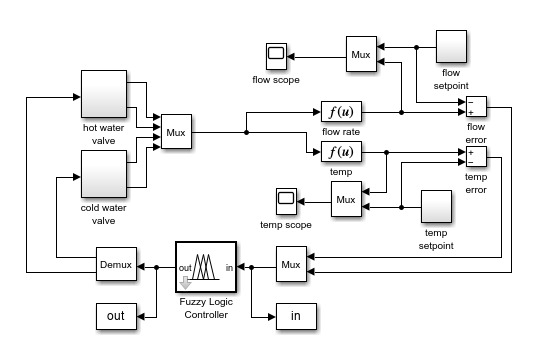For this system, you control the flow rate and temperature of a shower by adjusting hot and cold water valves.

Since there are two inputs for the fuzzy system, the model concatenates the input signals using a Mux block. The output of the Mux block is connected to the input of the Fuzzy Logic Controller block. Similarly, the two output signals are obtained using a Demux block connected to the controller.

### Fuzzy Inference System

The fuzzy system is defined in a FIS object, `fisMatrix`, in the MATLAB® workspace. For more information on how to specify a FIS in a Fuzzy Logic Controller block, see Fuzzy Logic Controller.

The two inputs to the fuzzy system are the temperature error, `temp`, and the flow rate error, `flow`. Each input has three membership functions.

```figure plotmf(fisMatrix,'input',1) figure plotmf(fisMatrix,'input',2) ```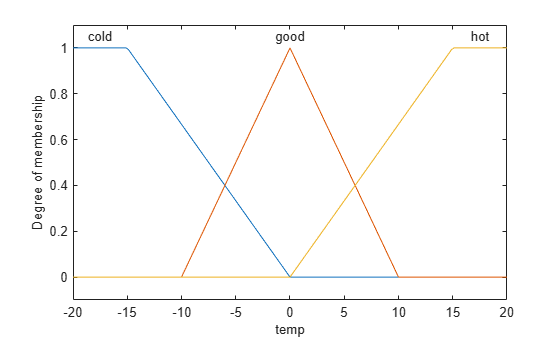The two outputs of the fuzzy system are the rate at which the cold and hot water valves are opening or closing, `cold` and `hot` respectively. Each output has five membership functions.

```figure plotmf(fisMatrix,'output',1) figure plotmf(fisMatrix,'output',2) ```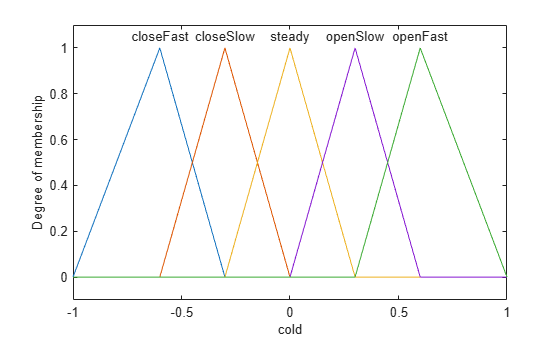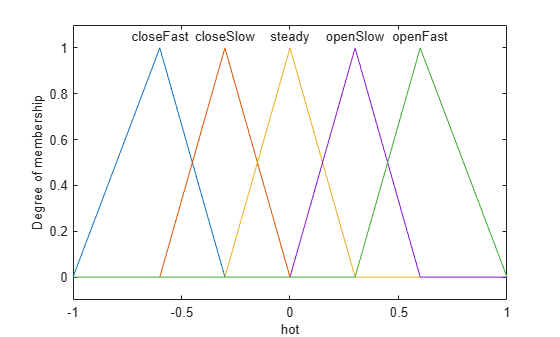The fuzzy system has nine rules for adjusting the hot and cold water valves based on the flow and temperature errors. The rules adjust the total flow rate based on the flow error, and adjust the relative hot and cold flow rates based on the temperature error.

```fisMatrix.Rules ```
```ans = 1x9 fisrule array with properties: Description Antecedent Consequent Weight Connection Details: Description ______________________________________________________________ 1 "temp==cold & flow==soft => cold=openSlow, hot=openFast (1)" 2 "temp==cold & flow==good => cold=closeSlow, hot=openSlow (1)" 3 "temp==cold & flow==hard => cold=closeFast, hot=closeSlow (1)" 4 "temp==good & flow==soft => cold=openSlow, hot=openSlow (1)" 5 "temp==good & flow==good => cold=steady, hot=steady (1)" 6 "temp==good & flow==hard => cold=closeSlow, hot=closeSlow (1)" 7 "temp==hot & flow==soft => cold=openFast, hot=openSlow (1)" 8 "temp==hot & flow==good => cold=openSlow, hot=closeSlow (1)" 9 "temp==hot & flow==hard => cold=closeSlow, hot=closeFast (1)" ```

### Simulation

The model simulates the controller with periodic changes in the setpoints of the water temperature and flow rate.

```set_param('shower/flow scope','Open','on','Ymin','0','Ymax','1') set_param('shower/temp scope','Open','on','Ymin','15','Ymax','30') sim('shower',50) ```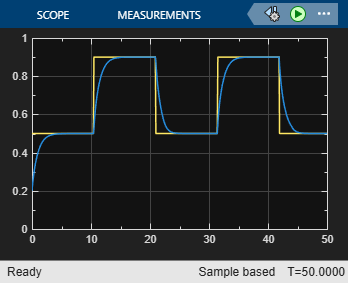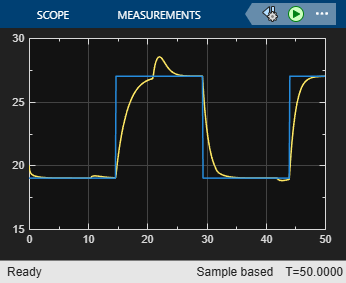The flow rate tracks the setpoint well. The temperature also tracks its setpoint, though there are temperature deviations when the controller adjusts to meet a new flow setpoint.

```bdclose('shower') % Closing model also clears its workspace variables. ```